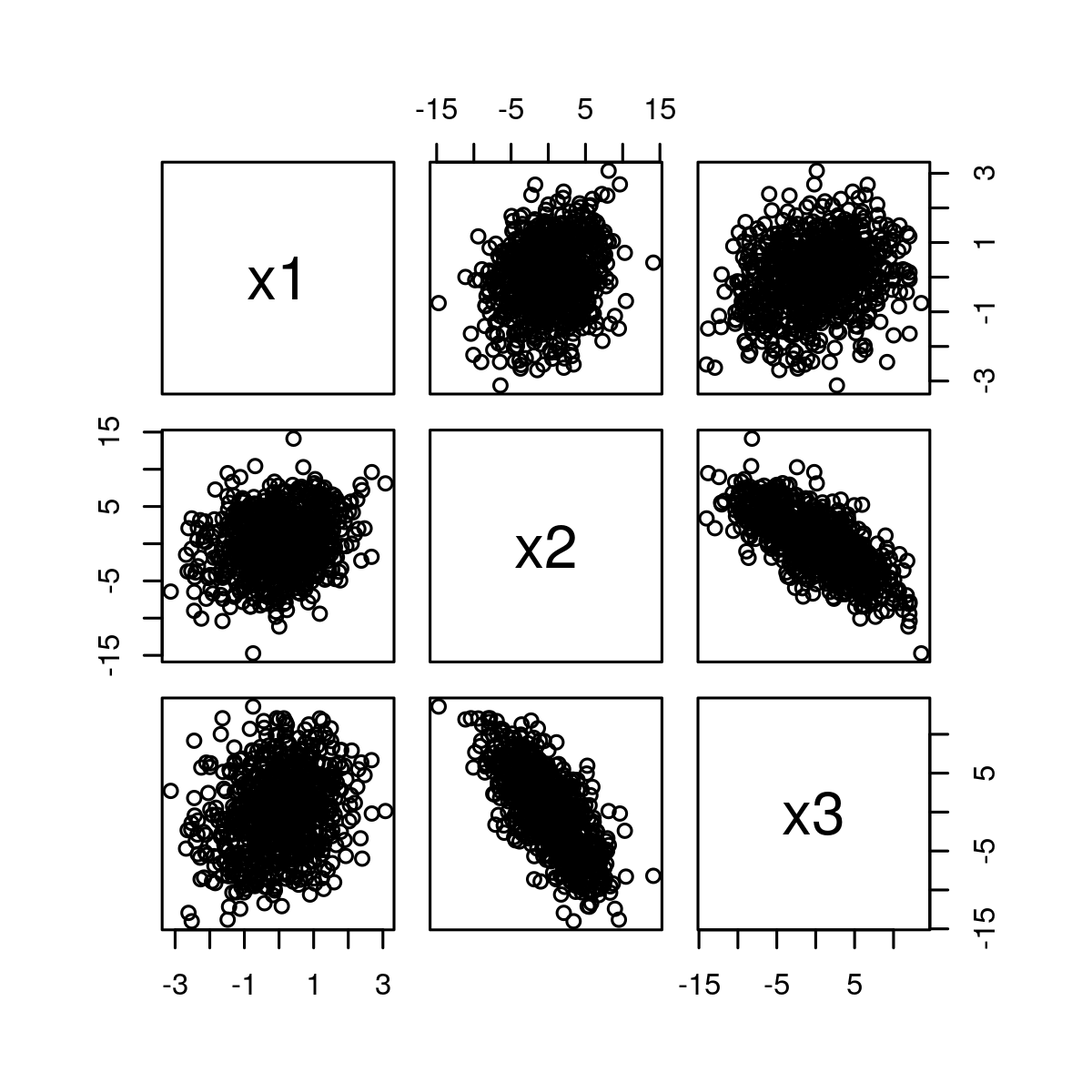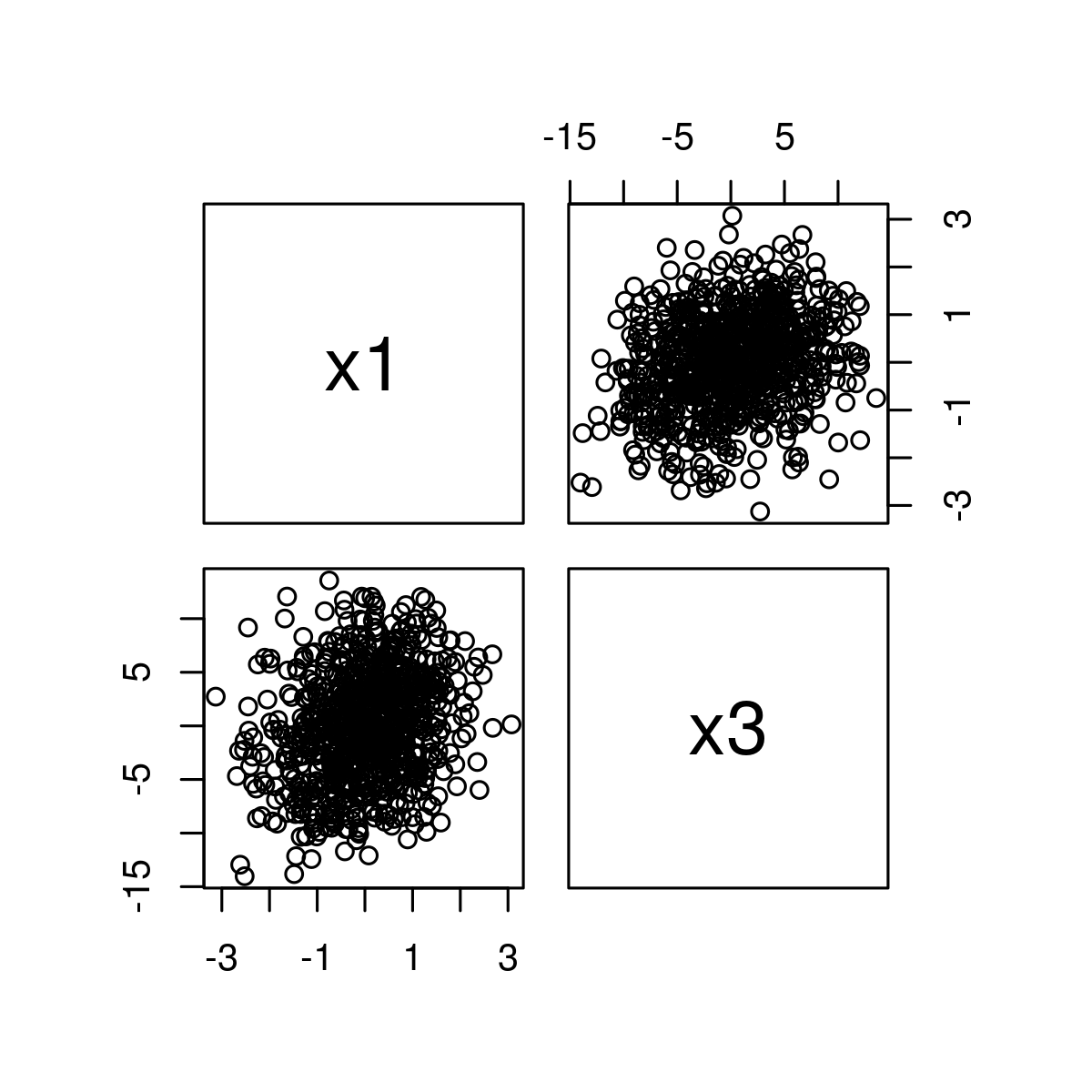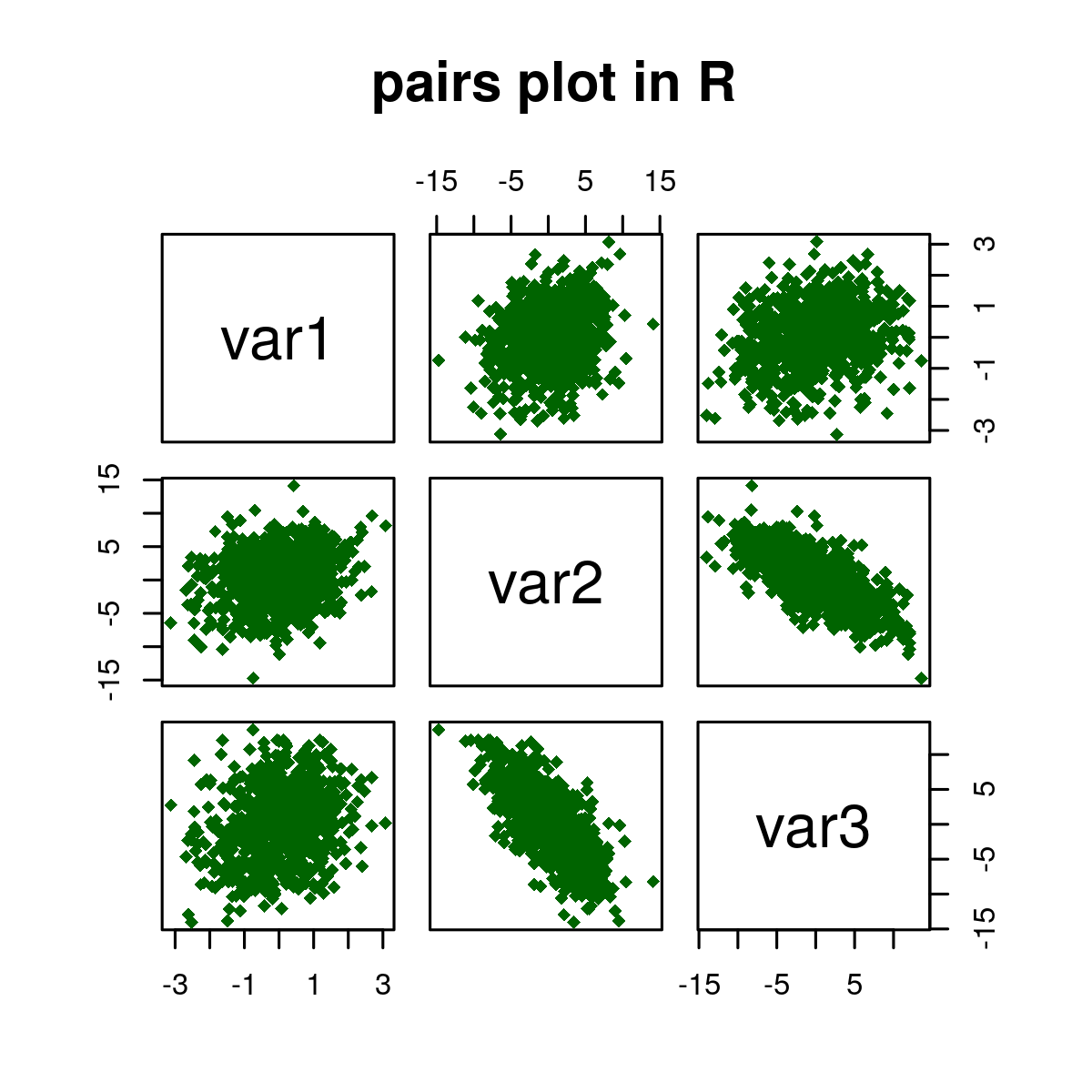# Create a Plot Matrix of Scatterplots in R Programming – pairs() Function

• Last Updated : 10 Dec, 2021

pairs() function in R language is used to return a plot matrix, consisting of scatter plots corresponding to each data frame.

## R – Create Plot Matrix of Scatterplots

Syntax: pairs(data)

Parameters:

• data: It is defined as  value of pairs Plot.

Returns: Color, Labels, Panels, and by Group in pairs plot.

## R

 `# Set seed for reproducibility``set.seed``(425340)` `# Sample size of 800``N <- 800` `# Create variable``x1 <- ``rnorm``(N)` `# Create correlated variable``x2 <- x1 + ``rnorm``(N, 0, 4)` `# Create another correlated variable``x3 <- 2 * x1 - x2 + ``rnorm``(N, 0, 3)   ` `data <- ``data.frame``(x1, x2, x3)   ``pairs``(data)           `

Output:Here, in the above example, the diagonal shows the names of the three numeric variables. The middle graphic in the first-row shows the relation between x1 and x2 whereas the right graph in the first row shows the relation between x1 and x3 and so on.

## R

 `# Set seed for reproducibility``set.seed``(425340)` `# Sample size of 800``N <- 800   ` `# Create variable``x1 <- ``rnorm``(N)` `# Create correlated variable``x2 <- x1 + ``rnorm``(N, 0, 4)           ` `# Create another correlated variable``x3 <- 2 * x1 - x2 + ``rnorm``(N, 0, 3)   ` `data <- ``data.frame``(x1, x2, x3)   ` `pairs``(~ x1 + x3, data = data)   `

Output:## R

 `# Set seed for reproducibility``set.seed``(425340)` `# Sample size of 800``N <- 800` `# Create variable``x1 <- ``rnorm``(N)` `# Create correlated variable``x2 <- x1 + ``rnorm``(N, 0, 4)` `# Create another correlated variable``x3 <- 2 * x1 - x2 + ``rnorm``(N, 0, 3)   ` `data <- ``data.frame``(x1, x2, x3)   ` `pairs``(~ x1 + x2 + x3, data = data)``pairs``(~ x1 + x3, data = data)   ``pairs``(data[, 1:3],``    ``col = ``"darkgreen"``, ``# Change color                   ``    ``pch = 18,             ``# Change shape of points``        ` `    ``# Change labels of diagonal``    ``labels = ``c``(``"var1"``, ``"var2"``, ``"var3"``),``    ``main = ``" pairs plot in R"``)`

Output:My Personal Notes arrow_drop_up